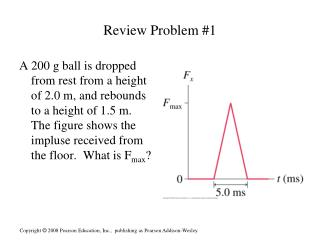DownloadDownload PresentationReview Problem #1

# Review Problem #1

Télécharger la présentation## Review Problem #1

- - - - - - - - - - - - - - - - - - - - - - - - - - - E N D - - - - - - - - - - - - - - - - - - - - - - - - - - -
##### Presentation Transcript

1. Review Problem #1 A 200 g ball is dropped from rest from a height of 2.0 m, and rebounds to a height of 1.5 m. The figure shows the impluse received from the floor. What is Fmax?

2. Review Problem #1 A 200 g all is dropped from rest from a height of 2.0 m, and rebounds to a height of 1.5 m. The figure shows the impluse received from the floor. What is Fmax? Fmax = 9.3 x 102 N (ignoring the impulse due to gravity)

3. Review Problem #2 The coefficient of static friction is 0.60 between the two blocks in the figure. The coefficient of kinetic friction between the lower block and the floor is 0.20. Force Fcauses both blocks to slide 5 meters, starting from rest. Determine the minimum amount of time in which the motion can be completed without the upper block slipping.

4. Review Problem #2 amax = 3.27 m/s2 tmin = 1.75 s

5. Review #3 A 60-gram ball is tied to the end of a 50-cm long string and swung in a vertical circle. The center of the circle, as shown in the figure, is 150 cm above the ground. The ball is swung at the minimum speed necessary to make it over the top without the string going slack. If the string is cut at the instant the ball is at the top of the loop, how far to the right does the ball hit the ground?

6. Review #3 – v = 2.21 m/s horizontally the time for the projectile to hit the ground is: t = .64 s x = 1.41 m from the point of release

7. Review Question #4 A 1 kg object has the potential energy shown in the graph • The object starts at rest at x = 0 m. What is its speed at x = 3 m? • What is its speed at x = 4 m? • Draw a force graph for this situation

8. Review Question #4 A 1 kg object has the potential energy shown in the graph • The object starts at rest at x = 0 m. What is its speed at x = 3 m? 1.4m/s • What is its speed at x = 4 m? 0 m/s • Draw a force graph for this situation

9. Review Problem #5 Santa parked his sleigh 5.0 m from the edge of a roof sloped at a 200 angle from horizontal. The reindeer went off on a smoke break. While they were gone, the parking brake failed. Santa’s sleigh slid down the roof and landed on the ground 6.0 m away from the wall of the house. The coefficients of friction between the sleigh and the snowy roof are: us = 0.1 and uk = 0.08. • what speed was the sled going when it fell off the roof? • How high was the roof?

10. Review Problem #5 Santa parked his sleigh 5.0 m from the edge of a roof sloped at a 200 angle from horizontal. The reindeer went off on a smoke break. While they were gone, the parking brake failed. Santa’s sleigh slid down the roof and landed on the ground 6.0 m away from the wall of the house. The coefficients of friction between the sleigh and the snowy roof are: us = 0.1 and uk = 0.08. • what speed was the sled going when it fell off the roof? 5.11 m/s • How high was the roof? 9.84 m

11. Guess my weight #6 • A rigid, 100 N board is placed across 2 bathroom scales separated by a distance of 2.00m. A person of that height lies on the board. The scale under his head reads 475 N and the scale under his feet reads 365 N. Find: a. his weight. • b. the position of the center of mass relative to his head.

12. Guess my weight • A rigid, 100-N board is placed across 2 bathroom scales separated by a distance of 2.00 m. A person of that height lies on the board. The scale under his head reads 475 N and the scale under his feet reads 365 N. Find: • a. his weight 740 N • b. his position of center of mass relative to his head 0.85 m

13. A student holds a meter stick horizontally. The number, mass and locations of the hanging objects vary. Rank the situations on the basis of how hard it would be for the student to keep the meter stick from rotating. Show ties. Explain your reasoning:

14. A student holds a meter stick horizontally. The number, mass and locations of the hanging objects vary. Rank the situations on the basis of how hard it would be for the student to keep the meter stick from rotating. Show ties. Explain your reasoning:

15. EOC # 54 • Find the position of the center of mass for this uniform plate with a cutout section.

16. EOC # 54 • Find the position of the center of mass for this uniform plate with a cutout section. • xcm = -.25 cm • ycm = .125 cm